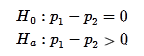## Hypothesis Test for Difference in Two Population Proportions (2 of 6)

### Learning Objectives

• Under appropriate conditions, conduct a hypothesis test for comparing two population proportions or two treatments. State a conclusion in context.
• Interpret the P-value as a conditional probability.

Before we get into the details of the hypothesis test for a difference in two population proportions, let’s review the general steps in hypotheses testing that we learned in Inference for One Proportion. The steps and the logic of the hypothesis test are the same as in that module. We also practiced this type of thinking more informally in the section “Distribution of Differences in Sample Proportions.”

Step 1: Determine the hypotheses.

The hypothesis comes from the research question. The null hypothesis is a statement of “no effect” or “no difference.” The alternative hypothesis reflects our claim.

Step 2: Collect the data.

Ideally, we select two independent random samples from two populations, or we randomly assign subjects to two treatments in an experiment.

Step 3: Assess the evidence.

We assume that the null hypothesis is true. This means we assume the population proportions are the same. Then we ask, Could the data come from populations with the same proportions? Now imagine taking random samples from these populations. Even if there is no difference between the population proportions, we expect variability in the differences between sample proportions. These differences are due to chance. We use simulation or a mathematical model to see how much the differences in sample proportions vary. Then we figure out if the difference we see in the data is likely or unlikely. Note that the wording “likely or unlikely” implies that this step requires some kind of probability calculation. We will again find a P-value. As before, the P-value is a probability related to the sampling distribution. It describes the chance that random samples will have a difference in sample proportions that is at least as extreme as we see in the data if the null hypothesis is true. “At least as extreme as” means as far from the center of the sampling distribution or further.

Step 4: State a conclusion.

We use the P-value to make a decision. The P-value helps us determine if the difference in proportions seen in the data is statistically significant or due to chance. One of two outcomes can occur.

• One possibility: The difference in sample proportions from the data is extremely unlikely. In this case, there is only a small chance that proportions from random samples differ more than what we observed in the data. So the probability (the P-value) is small, suggesting that the data did not come from populations with the same proportions. We view this as strong evidence against the null hypothesis. We reject the null hypothesis in favor of the alternative hypothesis.
• The other possibility: The difference in sample proportions observed in the data are fairly likely (not unusual). In this case, it is not surprising to see proportions from random samples with larger absolute differences than we observed in the data. The probability is large enough that we don’t think the data is unusual. It could come from populations with the same proportions. A large P-value suggests that we do not have evidence against the null hypothesis, so we cannot reject it in favor of the alternative hypothesis.

Before we get into the details of the hypothesis test, let’s practice using the P-value to make a decision.

### Learn By Doing

Recall the Abecedarian Project. In this experiment researchers randomly assigned infants to a treatment or control group. The treatment group received 5-years of high quality pre-school. We previously stated the following hypotheses to test the claim that a larger proportion of children who received the treatment will attend college.p1 is the the proportion of children who attend a quality preschool that eventually go to college.

p2 is the the proportion of children who did not attend a quality preschool that eventually go to college.